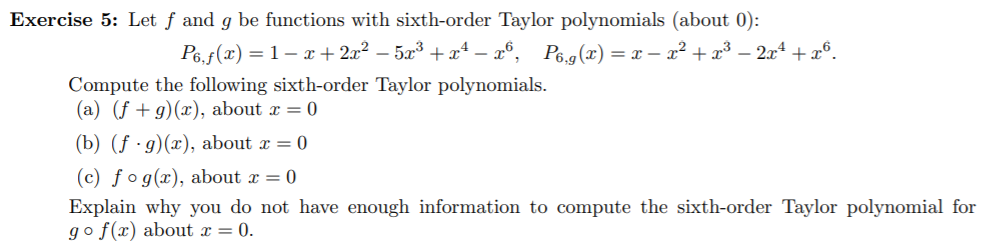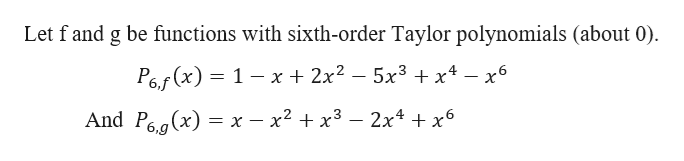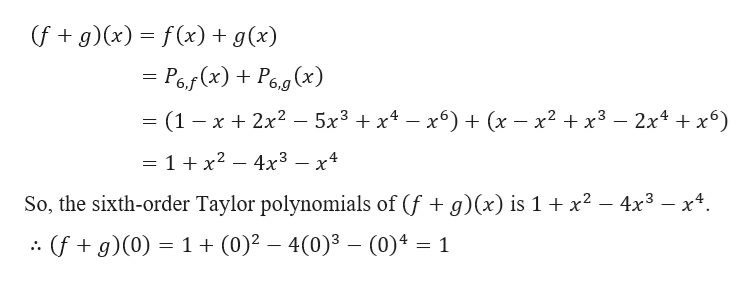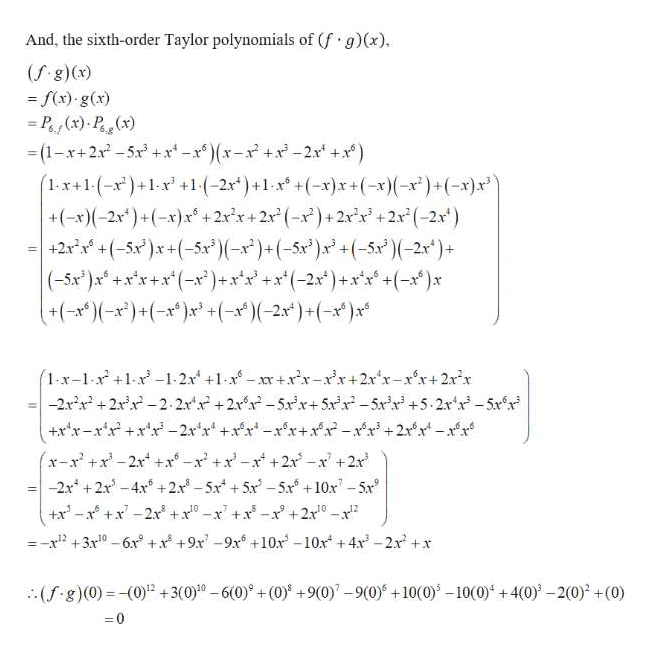# Exercise 5: Let f and g be functions with sixth-order Taylor polynomials (about 0):P6.f(x= 1-+2x2 -53 +x4 - 6,Pe.g(x) = x - x2+x3 -2x4 x6.Compute the following sixth-order Taylor polynomials(a) (f g) (x), about x = 0(b) (fg)(x), about x = 0(c) fog(x), about x = 0Explain why you do not have enough information to compute the sixth-order Taylor polynomial forgo f(x) about x = 0.

Question
16 viewshelp_outlineImage TranscriptioncloseExercise 5: Let f and g be functions with sixth-order Taylor polynomials (about 0): P6.f(x= 1-+2x2 -53 +x4 - 6, Pe.g(x) = x - x2+x3 -2x4 x6. Compute the following sixth-order Taylor polynomials (a) (f g) (x), about x = 0 (b) (fg)(x), about x = 0 (c) fog(x), about x = 0 Explain why you do not have enough information to compute the sixth-order Taylor polynomial for go f(x) about x = 0. fullscreen
check_circle

Step 1

Given,help_outlineImage TranscriptioncloseLet f and g be functions with sixth-order Taylor polynomials (about 0) 2x2 - 5x3 + x4 - x6 P6(x) = 1 - x And Pe.a(x) 3 х — х2 + х3 — 2x4 + х6 fullscreen
Step 2

Part ahelp_outlineImage Transcriptionclose(fg) (x) f(x) g(x) - Po(x)+P6g(x) 5x3x4-x6) + (x - x2 + x3- 2x4 + x6) (1 x 2x2 = 1 + x2 - 4x3 - x4 So, the sixth-order Taylor polynomials of (f + g)(x) is 1 x2 - 4x3 - x4 (fg)(0) 1(0)2 4(0)3- (0)4 1 fullscreen
Step 3

Part b

...help_outlineImage TranscriptioncloseAnd, the sixth-order Taylor polynomials of (f g)(x) (f g)(x) -f(x) -g(x) P(x) P () -(1-x+2x-5x+x-(x-x+x-2 +x) 1x+1(-x)+1x +1(-2x*)+1x + ( - x)x + (-x)(-x )+ ( -x)x +(-x)(-2x)+(-x)x+2xx+2x (-x)+ 2x+2x (-2x) +2xx +(-5x)x+(-5x*)(-x)+ (-5x )x + (-5x)( -2x)+ (-5x*)x +xx+x (-)+xx+x (-2x ) + xx+(-) +-x)+(xx+(--2x)+ ( -x )x (1-x-1-x +1x-1-2x+1x- xx + x-xx+2xx-xr+ 2xx -2xx+2x-2.2x +2-5xx+5x-5+5.2x x-5xx +xx-r -2x x +xx -x x+xx-x + 2xx -xx (x-x+x-2x +x-x +x-x +2x -x+2x -2x +2x-4x2x-5x +5x-5x +10x-5x +x -x +x-2x x1-x +x-x +2x-x 101 =- 2 + 3x10 -6x+x +9x-9x 10x -10x+4 -2x +x (f8)(0)- (0) +3(0-6(0)+(0) +9(0)-9(0 +10(0)-10(0)+4(0)-2(0) +(0) 0 fullscreen

### Want to see the full answer?

See Solution

#### Want to see this answer and more?

Solutions are written by subject experts who are available 24/7. Questions are typically answered within 1 hour.*

See Solution
*Response times may vary by subject and question.
Tagged in

### Calculus